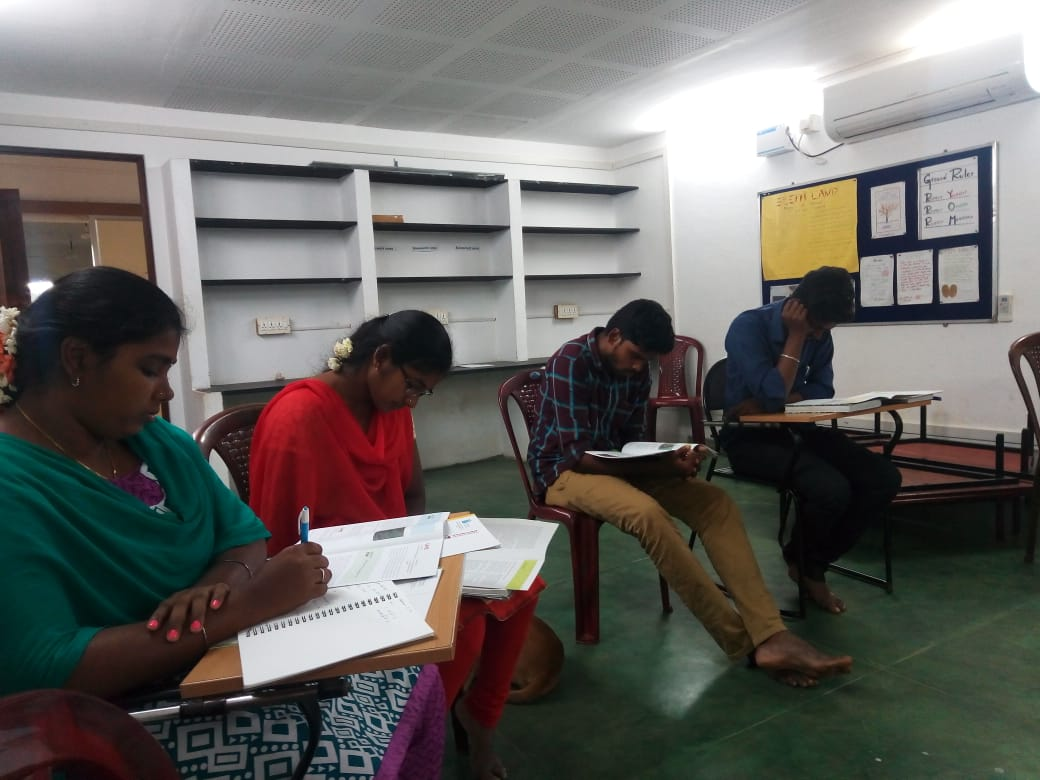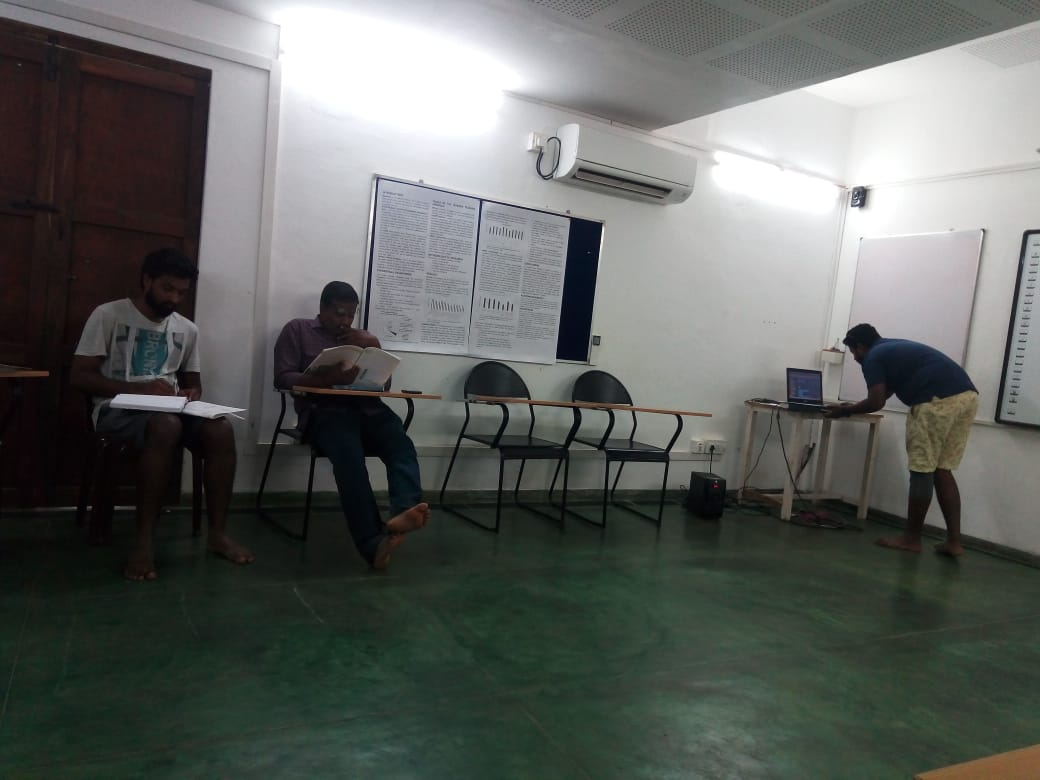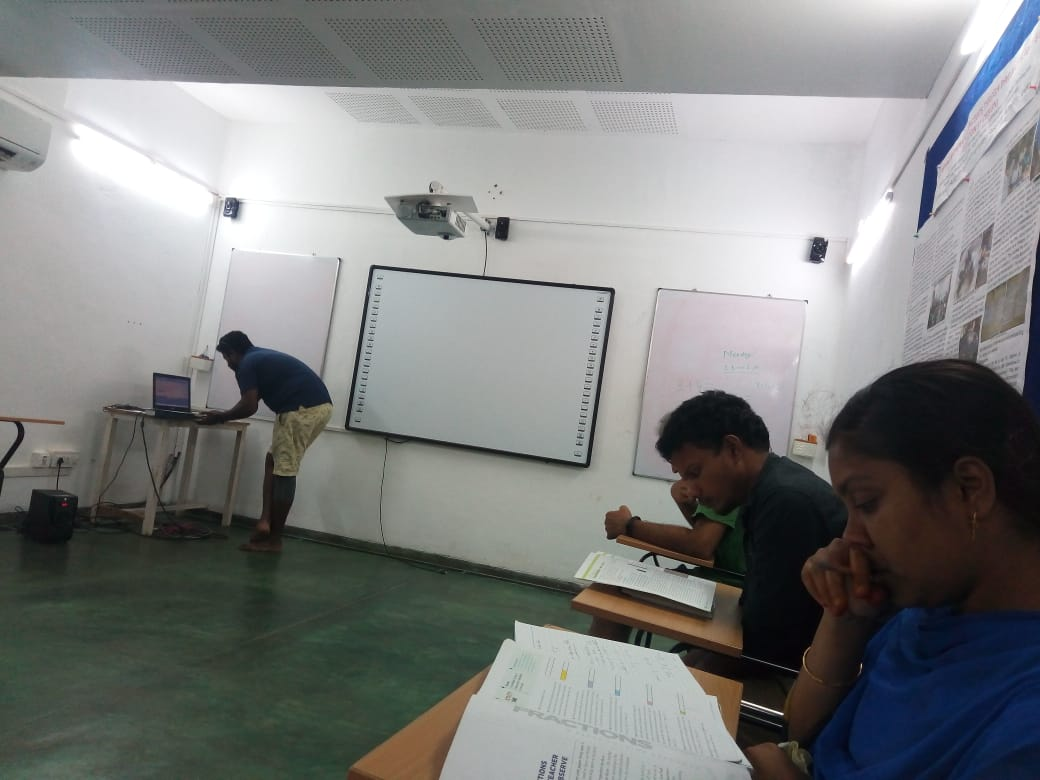# STEM land meeting

~Poovizhi, Abilash

Yesterday we had STEM land meeting with all the coordinators of different STEM land and STEM land math teachers. Sundar presented a scratch program for fraction. Later we were all given small booklets by Azim Premji University on each topic like Addition, subtraction, multiplication, measurement. It had different activities that could be done with children in learning the concept. We agreed to read the booklet and present what we learnt from it make a model of it and present it to others in the next session. For some time we all read our booklet in silence and took notes.Then shared about cyclic number which he read from his book which was new and interesting for all of us. The cyclic number is the following

1/7 = 0.142857

142857 x 2 = 285714

142857 x 3 = 428571

142857 x 4 = 571428

142857 x 5 = 714285

142857 x 6 = 857142

142857 x 7 = 999999

And the other one was to find the pattern and come up with an equation

1 = 1

3+5 = 8

7+9+11 = 27

What is the pattern and will the next line be?

1       = 1       : 1^3

3+5       = 8      : 2^3

7+9+11      = 27   : 3^3

13+ 15+17+19  = 64   : 4^3

n(n-1)+1 is the equation